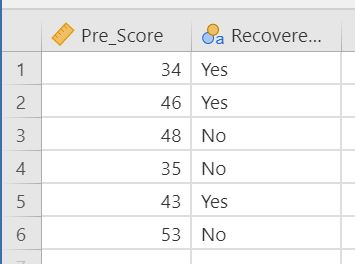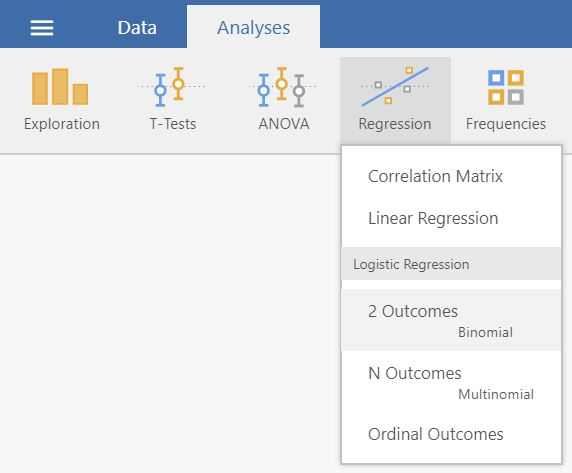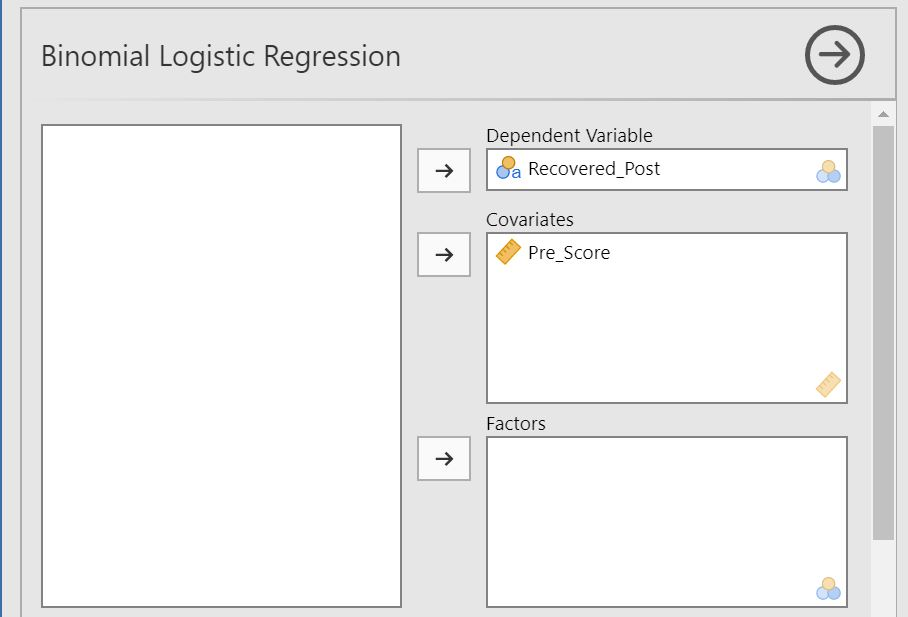Section author: Jonas Rafi

# Logistic Regression

How to perform a logistic regression in jamovi:
1. You need one categoricaldependent variable (nominal or ordinal), and at least one continuous explanatory variable.
A correct setup should look similar to this:2. Logistic regression can be found by selecting `Analyses``Regression`. If the outcome variable is nominal (as in the above image), select `2 Outcomes` if it has 2 steps / different values, or `N outcomes` if it has more than 2 steps. If the outcome variable is ordinal (e.g. low, medium, high), select `Ordinal Outcomes`.3. Drag and drop your dependent variable to `Dependent Variable` and your predictor to `Covariates`.4. The result is shown in the right panel:5. A little more comprehensive introduction into this statistical method is provided by this two videos, explaining logistic regression with two levels (to predict eg gender or clinical vs. control group ) and with more than two levels (to predict, e.g., food preferences: fast food, healthy food, high protein food, vegan food, etc.).